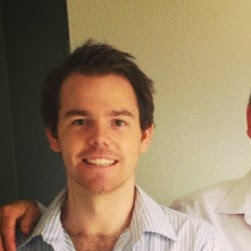Paul Rougieux - 2 years ago 301
R Question

# How to reproduce this moving distribution plot with R?

G Elliot Moris showed political polarization through time using a moving distribution plot.From this question: How to use 'facet' to create multiple density plot in GGPLOT I managed to use facets to reproduce a similar plot using dummy data:

``````library(ggplot2)
set.seed(101)
dtf <- data.frame(variable = c(rnorm(1000),
rnorm(1000) + rep(1:10/2,each =100)),
group = rep(c("a","b"), each = 1000),
year = rep(2001:2010, each=100))
ggplot(dtf) +
geom_density(aes(x = variable, fill = group)) +
facet_grid(year ~.)
``````But I would like the distributions to overlap as in the original plot. Is there a specific name for such plots and is it possible to reproduce them with R?

[EDIT] A dynamic version of a similar plot is available in this global temperature distribution plot.Ian

Update: the package used to created this plot is now named "ggridges".

You'll need the ggjoy package for this. Here's a rough version that needs some tidying up:

``````devtools::install_github("clauswilke/ggjoy")
library(ggjoy)
library(ggplot2)

#Create data frame
set.seed(101)
dtf <- data.frame(variable = c(rnorm(1000),
rnorm(1000) + rep(1:10/2,each =100)),
group = rep(c("a","b"), each = 1000),
year = rep(2001:2010, each=100))

# Use ggplot2 and ggjoy packages
ggplot(dtf,aes(x = variable, y = as.factor(year), fill = group)) +
geom_joy(scale = 2,alpha = .5,rel_min_height = 0.01) + theme_joy()
#> Picking joint bandwidth of 0.347
``````Recommended from our users: Dynamic Network Monitoring from WhatsUp Gold from IPSwitch. Free Download